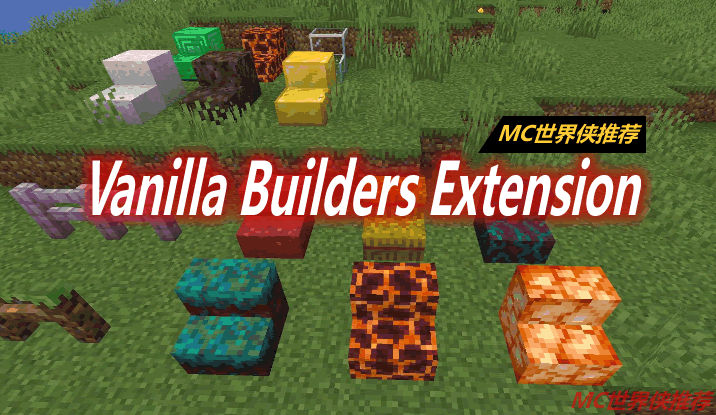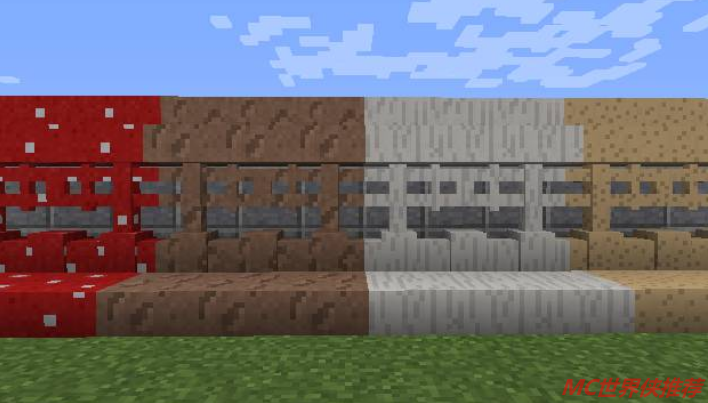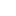# Vanilla Builders Extension Mod

Vanilla Builders Extension模组中添加了为原版所有缺失的添加了对应建筑，装饰方块的楼梯，台阶，栅栏，栅栏门和墙，添加的块使用其各自的原版材料的纹理，使它们能与每个材质包兼容。这样他们就可以处理每一个资源包。Vanilla Builders Extension Mod

• 古代碎片（仅1.16.4）。
• 玄武岩（仅1.16.4）。
• 蓝冰。
• 骨头。
• 凿石英。
• 凿红砂岩。
• 凿砂岩。
• 錾制石砖。
• 凿毛抛光黑石（仅1.16.4）。
• 凿制空心砖（仅1.16.4）。
• 粘土。
• 煤。
• 粗糙的泥土。
• 混凝土。
• 混凝土粉。
• 珊瑚。
• 碎砖（仅1.16.4）。
• 有裂纹的抛光黑石（仅适用于1.16.4）。
• 裂石砖。
• 哭泣的黑曜石（仅1.16.4）。
• 死珊瑚。
• 钻石。
• 污垢。
• 干海带。
• 翡翠的。
• 端石。
• 镀金黑石（仅1.16.4）。
• 玻璃。
• 黄金。
• 草。
• 砾石。
• 干草。
• 蜂蜜（仅1.15.2和1.16.4）。
• 蜂窝（仅1.15.2和1.16.4）。
• 菌丝（仅1.16.4）。
• 冰。
• 铁。
• 青金石。
• 树叶。
• 磁石（仅1.16.4）。
• 日志。
• 岩浆。
• 蘑菇。
• 菌丝体。
• 地狱疣。
• 尼日拉克。
• 网鲱鱼（仅1.16.4）。
• 尼龙（仅限1.16.4）。
• 黑曜石。
• 填充冰。
• 路径。
• 波达唑。
• 抛光玄武岩（仅1.16.4）。
• 紫柱。
• 石英砖（仅1.16.4）。
• 石英柱。
• 红砂。
• 红砖（仅1.16.4）。
• 红石。
• 沙子。
• Shroomlight（仅限1.16.4）。
• 粘液。
• 雪。
• 灵魂沙。
• 灵魂土壤（仅1.16.4）。
• 彩色玻璃。
• 阀杆（仅1.16.4）。
• 剥离菌丝（仅1.16.4）。
• 剥离的原木。
• 剥离阀杆（仅1.16.4）。
• 剥皮木。
• 目标（仅1.16.4）。
• 赤陶。
• 木头。
• 羊毛。

• 切割红砂岩。
• 切割砂岩。
• 光滑的石头。

• 暗宝石色。
• 磨制安山岩。
• 磨制闪长岩。
• 磨制花岗岩。
• 棱柱砖。
• 紫色。
• 石英。
• 光滑石英。
• 光滑红砂岩。
• 平滑沙石。
• 石头。

• 安山岩。
• 黑石（仅1.16.4）。
• 砖。
• 鹅卵石。
• 闪长岩。
• 端头石砖。
• 花岗岩。
• 苔石。
• 苔藓石砖。
• 抛光黑石（仅1.16.4）。
• 抛光黑石砖（仅1.16.4）。
• 普里斯马林。
• 红砖。
• 红砂岩。
• 砂岩。
• 石砖。
• 地下砖块。1.16.4

1.15.2

1.14.4

1.12.2

1.11.2